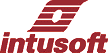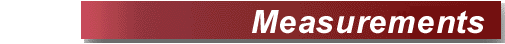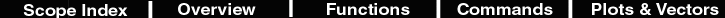Average
 Description Displays the mean value of current waveform between Cursors 0 and 1. The currently selected waveforms vector is integrated between cursor 0 and 1 and the result is divided by the interval between cursors to obtain the average value. The result is reported in the accumulator. Hot Key a Script loadaccumulator average(current) "Mean" Example Open scope and paste this script into the command window. Then press to plot a sine wave. Press to see the average value; notice that accuracy improves as numpoints is increased. set units = rad pi =4*atan(1) numpoints = 1000 time = (vector(numpoints)/(numpoints-1)*1m) newplot sineplot time setplot sineplot y = sin(2*pi*time*1k) plot y homecursors setcursor 1 500u set units = deg print 2/pi

Data Points
 Description Number of data points in the current waveform. Hot Key # Script loadaccumulator length(current) "Data Points" Example Open scope and paste this script into the command window. Then press to plot a sine wave. Select Measurements/Data Points in the calculator menu to show the number of points in the sine wave. set units = rad pi =4*atan(1) numpoints = 1000 time = (vector(numpoints)/(numpoints-1)*1m) newplot sineplot time setplot sineplot y = sin(2*pi*time*1k) plot y homecursors set units = deg print numpoints

Maximum
 Description Maximum value of current waveform between Cursors 0 and 1. Hot Key > Script loadaccumulator max(current) "Maximum" Example Open scope and paste this script into the command window. Then press to plot a sine wave. Select Measurements/Maximum in the calculator menu to evaluate the maximum value of the sine wave. set units = rad pi =4*atan(1) numpoints = 1000 time = (vector(numpoints)/(numpoints-1)*1m) newplot sineplot time setplot sineplot y = sin(2*pi*time*1k) plot y homecursors set units = deg echo "max = 1"

Minimum
 Description Minimum value of current waveform between Cursors 0 and 1. Hot Key < Script loadaccumulator min(current) "Minimum" Example Open scope and paste this script into the command window. Then press to plot a sine wave. Select Measurements/Minimum in the calculator menu to evaluate the minimum value of the sine wave. set units = rad pi =4*atan(1) numpoints = 1000 time = (vector(numpoints)/(numpoints-1)*1m) newplot sineplot time setplot sineplot y = sin(2*pi*time*1k) plot y homecursors set units = deg echo "min = -1"

Parametric Cursor
 Description Makes the current trace a master trace and creates a linked parametric plot and trace with auto-labels linked to the master trace and cursor 1. This makes it possible to analyze curve families, such as those created by a DC sweep. The currently selected waveforms vector is integrated between cursor 0 and 1 and the result is divided by the interval between cursors to obtain the average value. The result is reported in the accumulator. Hot Key none Script none Example Run SpiceNet Open "\Spice8\Circuits\Examples\DCSweep.DWG" Select Simulate in the Actions menu Run Scope5 Select "i_v2" in the Add Waveform dialog and click Add Select Measurements\ParametricCursor in the Calculator menu Drag Cursor 1 in Plot2, and watch the auto-labels in Plot1 and track the cursor movement.

Peak to Peak
 Description Difference between max and min values of current waveform between Cursors 0 and 1. Hot Key p Script loadaccumulator pk_pk(current) "Peak to Peak" Example Open scope and paste this script into the command window. Then press to plot a sine wave. Select Measurements/Peak to Peak in the calculator menu to evaluate the pk-pk value of the sine wave. set units = rad pi =4*atan(1) numpoints = 1000 time = (vector(numpoints)/(numpoints-1)*1m) newplot sineplot time setplot sineplot y = sin(2*pi*time*1k) plot y homecursors set units = deg echo "pk-pk = 2"

RMS
 Description RMS of current waveform between Cursors 0 and 1. Hot Key r Script loadaccumulator rms(current) "RMS"d 1. Example Open scope and paste this script into the command window. Then press to plot an offset sine wave Select Measurements/RMS in the calculator menu to evaluate the rms value of the sine wave. set units = rad pi =4*atan(1) numpoints = 1000 time = (vector(numpoints)/(numpoints-1)*1m) newplot sineplot time setplot sineplot y = sin(2*pi*time*1k) + 1 plot y homecursors set units = deg rms = sqrt(1+.5) print rms

Std Deviation
 Description Standard deviation (AC RMS) of current waveform between Cursors 0 and 1. The average value is removed from the current waveform, then the RMS is computed. Hot Key \$ Script loadaccumulator stddev(current) "Std. Deviation" Example Open scope and paste this script into the command window. Then press to plot an offset sine wave. Select Measurements/RMS in the calculator menu to evaluate the rms value of the sine wave. set units = rad pi =4*atan(1) numpoints = 1000 time = (vector(numpoints)/(numpoints-1)*1m) newplot sineplot time setplot sineplot y = sin(2*pi*time*1k) + 1 plot y homecursors set units = deg print StdDev=sqrt(.5)

X at Maximum
 Description X at maximum value of current waveform between Cursors 0 and 1. Hot Key M Script temp = getcursorx(0) movecursorright(0, current, max(current)) loadaccumulator getcursorx(0) "X at Maximum" setcursor(0, temp) Example Open scope and paste this script into the command window. Then press to plot an offset sine wave. Select Measurements/X at Maximum in the calculator menu find the x axis coordinate of the maximum value. set units = rad pi =4*atan(1) numpoints = 1000 time = (vector(numpoints)/(numpoints-1)*1m) newplot sineplot time setplot sineplot y = sin(2*pi*time*1k) + 1 plot y homecursors set units = deg

X at Minimum
 Description X at minimum value of current waveform between Cursors 0 and 1. Hot Key m Script temp = getcursorx(0) movecursorright(0, current, min(current)) loadaccumulator getcursorx(0) "X at Minimum" setcursor(0, temp) Example Open scope and paste this script into the command window. Then press to plot an offset sine wave. Select Measurements/X at Minimum in the calculator menu find the x axis coordinate of the minimum value. set units = rad pi =4*atan(1) numpoints = 1000 time = (vector(numpoints)/(numpoints-1)*1m) newplot sineplot time setplot sineplot y = sin(2*pi*time*1k) + 1 plot y homecursors set units = deg